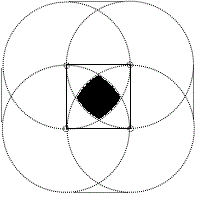BrainDen.com - Brain Teasers

## Question

There is a unit square on a plane. A unit circle (r=1) is placed randomly, so that its center is somewhere inside the unit square. Every point inside the unit square has the same probability of being chosen as the center of the unit circle.

What is the probability that the square fits entirely inside the circle?

## Recommended Posts

• 00?

##### Share on other sites

• 0

Did some drawing and I came up with 31.51%. No time to show reasoning now, but that's my best guess. Area looks like a square made with 4 arc segments, each radius 1, with centers on corners of the square.

##### Share on other sites

• 0Did some drawing and I came up with 31.51%. No time to show reasoning now, but that's my best guess. Area looks like a square made with 4 arc segments, each radius 1, with centers on corners of the square.

You went further than I did, but sketching it out real quick like, that's what I get. Your prob passes my reasonability test.

##### Share on other sites

• 0Did some drawing and I came up with 31.51%. No time to show reasoning now, but that's my best guess. Area looks like a square made with 4 arc segments, each radius 1, with centers on corners of the square.

I came up with a similar drawing as you did but I got a slightly different answer

~34.31%

I'm working on trying to make it more accurate.

##### Share on other sites

• 01 - [sQRT(3) - Pi/3] = ~ .3151467436

could you explain?

##### Share on other sites

• 0probability is (3-2*SQR2)2

##### Share on other sites

• 0could you explain?

Refer to the attached image

spvu is the unit square

Capital letters denote area of the enclosed region and small letters are references for points

Now, the area you want to calculate for probability is O; ie as long as the centre of cirle is in this region, the square is inside the cricle completely

Now, consider triangle pqr.

pq = 1 and rp = 1/2

this means qr = root(3) /2

Area of triangle pqr = root(3) / 8

Also angle subtended by arc qst at p is 120° as angle qpr = 60°

so area of region qstp = pi/3

Now area of qstr = area of arc qstp - 2*area of trianlge pqr

So, area of qstr = pi/3 - root(3) / 4

And by symmetry, this is also the area enclosed in region by points spt

Now area of arc sptu = pi/4

And area of square spuv = 1

So, area of region puv = G+C+H = 1 - pi/4

Now area of D+O+B = area of spu - (E+A+F)

By symmetry, E+A+F = G+C+H

So, area of D+O+B = pi/4 - (1-pi/4) = pi/2 - 1

Now, A+O+B+F = area of spt

D+O+B = A+O+B

So, F = spt - (A+O+B)

F = (1 - root(3) /4) - (pi/6)

Now, A = pvu - 2F

Solving it you get

A = pi/12 - 1 + root(3) / 2

Now area of O = AOB - 2A

O = pi/2 - 1 - 2A

Solving it you get

O = 1 - root(3) + pi/3

O = 0,3151

Square and circles.bmp

Edited by DeeGee
##### Share on other sites

• 0There is a unit square on a plane. A unit circle (r=1) is placed randomly, so that its center is somewhere inside the unit square. Every point inside the unit square has the same probability of being chosen as the center of the unit circle.

What is the probability that the square fits entirely inside the circle?

38.45 %. The shadowed area in the attached draw, is the square region that accomplish condition. It is easy calculate the proportion of total doing it in a quarter. I could send a detailed reasoning.Edited by Jalegre
##### Share on other sites

• 038.45 %. The shadowed area in the attached draw, is the square region that accomplish condition. It is easy calculate the proportion of total doing it in a quarter. I could send a detailed reasoning.

Sorry the solution is the former posted, I had a calculus error.

## Join the conversation

You can post now and register later. If you have an account, sign in now to post with your account.×   Pasted as rich text.   Paste as plain text instead

Only 75 emoji are allowed.

×   Your previous content has been restored.   Clear editor

×   You cannot paste images directly. Upload or insert images from URL.